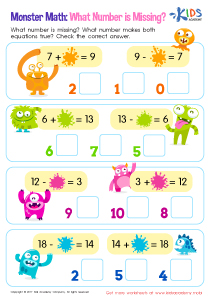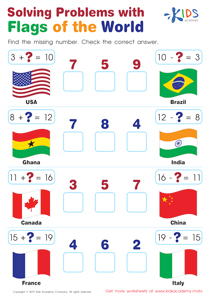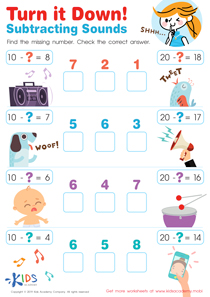# Math Lesson - Using Unknown addend problems to help with Subtraction Part 2, Grade 1

• ### Activity 1 / Missing Number: Monster Math WorksheetMonsters, maths and fun has a nice ring to it, don’t you think? The worksheet requires your child use their counting, addition and subtraction skills. There are equations on both sides of the page. The equation on the left is an addition problem, while the equation on the left mirrors the equation in the subtraction form. Help your child figure out which number out of the options provided makes both the equations true.

• ### Activity 2 / Solving Problems with Flags of the World WorksheetIn the world, there are many different countries. Some of these countries are very far away from each other, while some are very close to each other, and even share some areas of land. Ask your kids if they can name some of the countries they are familiar with. Now, introduce them to the flags of these countries using this simple worksheet. Help them find the missing number in each addition problem, and then check the correct answer.

• ### Activity 3 / Turn It down! Subtracting Sounds WorksheetIf your kids are struggling with mathematics in school, or you just feel like they could really use some extra help with their subtraction skills, then this worksheet is just what you need. The math problems your kids face in school will not be enough to help them fully understand mathematics. With some one-on-one time with you, they will enjoy the subject even more. In this worksheet, your kids will need to find the missing number in all the questions. Help them check the correct answer.

?